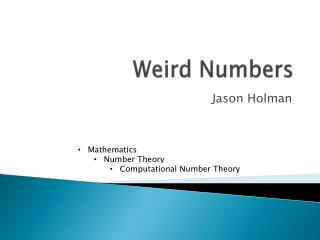DownloadDownload PresentationWeird Numbers

# Weird Numbers

Download Presentation## Weird Numbers

- - - - - - - - - - - - - - - - - - - - - - - - - - - E N D - - - - - - - - - - - - - - - - - - - - - - - - - - -
##### Presentation Transcript

1. Weird Numbers Jason Holman • Mathematics • Number Theory • Computational Number Theory

2. Types of number to be discussed • Abudnant • Deficient • Perfect • Pseudoperfect

3. Abundant • An integer is abundant if the sum of its proper divisors (all except the number itself) is greater than the number • Examples • 18, 24

4. Deficient • An integer is deficient if the sum of its proper divisors is less than the number • Examples • 35, 50, primes

5. Perfect • An integer is perfect if the sum of its proper divisors is equal to the number • Examples • 6, 28

6. Pseudoperfect • An integer is pseudoperfect if a subset of its proper divisors is equal to the number • Examples • 18, 24

7. Definition of weird number • A number is weird if it is abundant but not pseudoperfect

8. Examples of weird numbers • The first few weird numbers are 70, 836, 4030, 5830, 7192, 9272 • Example of how 70 is weird

9. Big picture problem • No known odd weird numbers • The weird numbers known so far have been computed either manually or using a computer • It is believed that an equation would help find the answer to this problem, but one has not been found • So the answer to this problem currently relies on extremely tedious computations

10. Sources • http://blogs.ams.org/mathgradblog/2013/08/29/odd-weird-numbers/ • http://mathworld.wolfram.com/WeirdNumber.html